Ch 9. 3-D Motion of a Rigid Body Multimedia Engineering Dynamics Translating Coordinates Rotating Coordinates Inertia & Ang. Momentum Equationsof Motion
 Chapter - Particle - 1. General Motion 2. Force & Accel. 3. Energy 4. Momentum - Rigid Body - 5. General Motion 6. Force & Accel. 7. Energy 8. Momentum 9. 3-D Motion 10. Vibrations Appendix Basic Math Units Basic Equations Sections Search eBooks Dynamics Fluids Math Mechanics Statics Thermodynamics Author(s): Kurt Gramoll ©Kurt GramollDYNAMICS - THEORY

Chapter 6 introduced moment of inertia to describe the angular motion of a rigid body in a plane. To analyze motion in 3-D, moments of inertia is still used but an additional term, product of inertia, must also be determined.

Moment of Inertia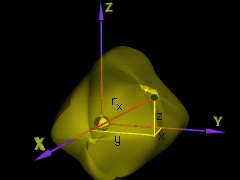Coordinate System

Recall, the moment of inertia of the element dm about the x axis is defined as

dIxx = rx2 dm     (noting rx2 = y2 + z2)

Integrating over the mass, gives,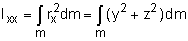Thus, all three directions are(1a)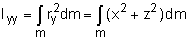(1b)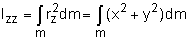(1c)

Product of Inertia

The product of inertia of the element dm is defined in relation to a set of two orthogonal planes as the product of the mass of the element and the perpendicular distance from the planes to the element.

For example, the product of inertia of an element with respect to the x-z and y-z planes is

dIxy = xy dm

Integrating over the mass, gives,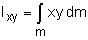For all three directions are(2a)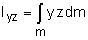(2b)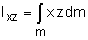(2c)

Parallel Axis and Parallel Plane Theorems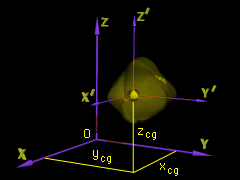Center of Gravity Dimensions

If the center of gravity of the body is located at xcg, ycg, zcg within the xyz system, the parallel axis equations are

 Ixx = (Ix'x')cg + m(ycg2 + zcg2)          (3a) Iyy = (Iy'y')cg + m(xcg2 + zcg2)          (3b) Izz = (Iz'z')cg + m(xcg2 + ycg2)           (3c)

Using a similar analysis, the parallel plane equations can be derived that transfer the products of inertia from a set of orthogonal planes passing through the body's center of gravity to a parallel set of planes passing through some other point O,

 Ixy = (Ix'y')cg + mxcgycg                 (4a) Iyz = (Iy'z')cg + mycgzcg                (4b) Ixz = (Ix'z')cg + mxcgzcg                (4c)

Angular Momentum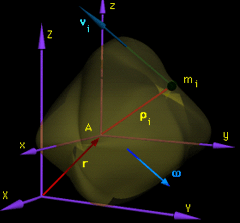Angular Acceleration Dimensions

Consider a rigid body moving in general motion relative to an inertial reference frame XYZ. The origin A of the xyz axes translates and rotates with the rigid body, which has an angular velocity ω relative to XYZ.

Now find the angular momentum of the body about point A relative to XYZ. The angular momentum of the ith particle, with mass mi, about point A is

HA-i = ρi/A × mivi

where ρi/A represents the position vector from A to the mass element, mi. For simplicity, it will be noted as ρi. Recall, the velocity vi is given by

vi = vA + ω × ρi

Substituting and integrating over the mass, gives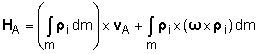If A is located at the center of gravity, the first term in H is zero. This gives,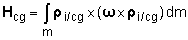(5)

If A is a fixed point O about which the mass rotates, vA = 0, then it is similar to the cg form,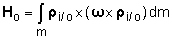(6)

If A is an arbitrary point on the body, the total angular momentum reduces to

 HA = ρcg/A × mvcg + Hcg                 (7)

Note that Eqs. 5, 6, and 7 all contain the general form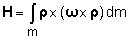If the coordinate system is rectangular (xyz), then the three components are,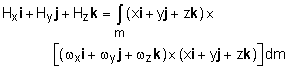Expanding the cross products, combining terms, and making use of Eqs. 1, the angular momentum for xyz coordinate system is

 Hx = Ixxωx + Ixyωy + Ixzωz                 (8a) Hy = Iyxωx + Iyyωy + Iyzωz                 (8b) Hz = Izxωx + Izyωy + Izzωz                 (8c)

These represent the scalar forms of Eqs. 5 or 6.

Practice Homework and Test problems now available in the 'Eng Dynamics' mobile app
Includes over 400 problems with complete detailed solutions.
Available now at the Google Play Store and Apple App Store.